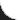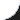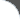Home | Tutorials | Articles | Videos | Products | Tools | Search
 Interviews | Open Source | Tag Cloud | Follow Us | Bookmark | Contact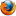In Browser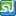StumbleUpon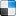del.icio.usGoogle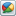Google Buzz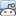redditLinkedInFacebook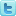TwitterLinkedinE-Mail
 Java > Collection > Maps > Map Manipulation

# Map Manipulation

The following example shows Map data manipulation. HashMap and Hashtable are two implementations of Map interface. Hashtable is synchronized where as HashMap is not. Map provides the following APIs,

```public int size();
- Returns the number of entries in the Map.

public boolean isEmpty();
- Returns true if the Map contains more than one entry otherwise returns false.

public V put(K paramK, V paramV);
- Puts a key value pair as an entry in Map.

public void putAll(Map<? extends K, ? extends V> paramMap);
- Copies all Map entries from specified Map.

public V remove(Object paramObject);
- Removes the specified entry from Map.

public void clear();
- Removes all entries from Map.
```

 File Name  : com/bethecoder/tutorials/utils/maps/ManipulateMap.java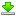Author  : Sudhakar KV Email  : [email protected]

 ``` package com.bethecoder.tutorials.utils.maps; import java.util.HashMap; import java.util.Map; public class ManipulateMap {   /**    * @param args    */   public static void main(String[] args) {     Map numMap = new HashMap();     System.out.println("Is Map Empty ? : " + numMap.isEmpty());          numMap.put(new Integer(1), "ONE");     numMap.put(new Integer(2), "TWO");     numMap.put(new Integer(3), "THREE");          System.out.println("Map Size : " + numMap.size() + " " + numMap);     System.out.println("Is Map Empty ? : " + numMap.isEmpty());     System.out.println();     //Copy all map entries     Map numMap2 = new HashMap();     numMap2.put(new Integer(4), "FOUR");     numMap2.put(new Integer(6), "SIX");          numMap.putAll(numMap2);     System.out.println("Map Size : " + numMap.size() + " " + numMap);     System.out.println("Is Map Empty ? : " + numMap.isEmpty());     System.out.println();          //Remove a particular key     numMap.remove(new Integer(1));     System.out.println("Map Size : " + numMap.size() + " " + numMap);     System.out.println("Is Map Empty ? : " + numMap.isEmpty());     System.out.println();          //Clear all map entries     numMap.clear();     System.out.println("Map Size : " + numMap.size() + " " + numMap);     System.out.println("Is Map Empty ? : " + numMap.isEmpty());        } }```

It gives the following output,
```Is Map Empty ? : true
Map Size : 3 {1=ONE, 2=TWO, 3=THREE}
Is Map Empty ? : false

Map Size : 5 {1=ONE, 2=TWO, 3=THREE, 4=FOUR, 6=SIX}
Is Map Empty ? : false

Map Size : 4 {2=TWO, 3=THREE, 4=FOUR, 6=SIX}
Is Map Empty ? : false

Map Size : 0 {}
Is Map Empty ? : true
```